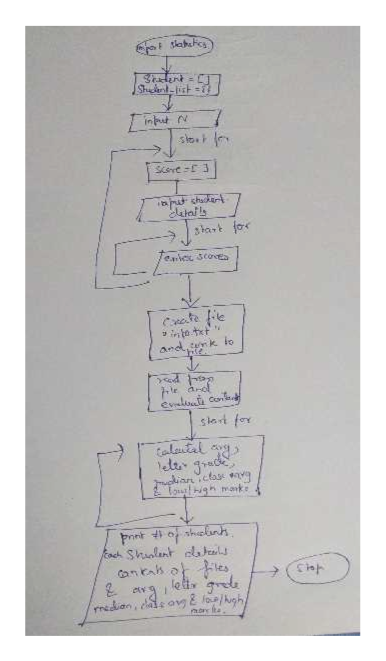# Hello, please I need help with the following using Python:  Write flowchart, pseudo-code and program for the following requirements, need to show written file content, and all results.A class of N students (N determined at runtime by user) with 10 grades. Need to input student 1st and last name, ID and scores, and write the information into a file.Read the file, calculate each student’s average score, letter grade, # of students (N), median score for all students, class average, who has the highest/lowest score.Print content of file and all results.Use a couple of students and 3 or 4 grades for testing the code first.

Question
32 views

Hello, please I need help with the following using Python:

Write flowchart, pseudo-code and program for the following requirements, need to show written file content, and all results.

A class of N students (N determined at runtime by user) with 10 grades. Need to input student 1st and last name, ID and scores, and write the information into a file.

Read the file, calculate each student’s average score, letter grade, # of students (N), median score for all students, class average, who has the highest/lowest score.

Print content of file and all results.Use a couple of students and 3 or 4 grades for testing the code first.

check_circle

Step 1

Pseudocode:

1. Import module statistics and Declare variables Students and Student_list
2. Prompt for the number of students
3. For each student:
• Prompt for first name, last name ID
• Prompt for scores and input ten grade scores.
• Save the details in Student_list and append each Student_list into
4. Create a text file "info.txt" and save the details of students into it
5. Open the saved file and read from it.
6. Print the contents of the file.
7. Declare Students_avg_scores, min_sc and max_sc
8. Print the total number of students
9. For each student:
• calculate the average score and print it
• Find the letter grade and print it
• Find the median and print it
10. Print the class average and the name of students with the highest and lowest marks.
Step 2

Program:

import statistics

Students = []

Student_list = {}

N = int(input("Please enter the number of students:"))

for i in range(N):

scores = []

print(" Enter details of Student",i+1,":")

first_name = input("Enter the first name of the student:")

last_name = input("Enter the last name of the student:")

ID = input("Enter the ID name of the student:")

print("Enter the scores:")

for j in range(10):

score = float(input("Enter the score of the student:"))

scores.append(score)

Student_list = {"first_name":first_name,

"last_name":last_name,

"ID" : ID,

"scores": scores

}

Students.append(Student_list)

f = open("info.txt","w")

f.write( str(Students) )

f.close()

print(" Contents of the file:")

print(f)

Student_info = eval(f)

Students_avg_scores = []

min_sc = 100

max_sc = 0

print(" Total number of students:",N)

for i in range(N):

sc= Student_info[i].get("scores")

avg_sc = sum(sc) / len(sc)

print(" Average score of Student",i+1," :",avg_sc)

if min_sc>avg_sc:

min_sc = avg_sc

min_index = i

if max_sc<avg_sc:

max_sc = avg_sc

max_index = i

if avg_sc >= 90: letter_grade = "A"

elif avg_sc >= 80: letter_grade = "B"

elif avg_sc >= 70: letter_grade = "C"

elif avg_sc >= 60:letter_grade = "D"

median = statistics.median(sc)

print("Median score of Student",i+1,":",median)

Students_avg_scores.append(avg_sc)

class_avg = sum(Students_avg_scores) / len(Students_avg_scores)

print(" Class average:",class_avg)

print(Student_info[min_index].get("first_name"), "has the lowest marks")

print(Student_info[max_index].get("first_name"), "has the highest marks")

Step 3

Flowchart:

...help_outlineImage TranscriptioncloseShackent it slo shd eatalls hak for eaieu scoes tt and, k io slot for talutal dianictase n ogh mak sheanh Print och Shialant ca coneah ot les arglettsgl nedion, eavy lo/gh 4 nor ta fullscreen

### Want to see the full answer?

See Solution

#### Want to see this answer and more?

Solutions are written by subject experts who are available 24/7. Questions are typically answered within 1 hour.*

See Solution
*Response times may vary by subject and question.
Tagged in

### Python